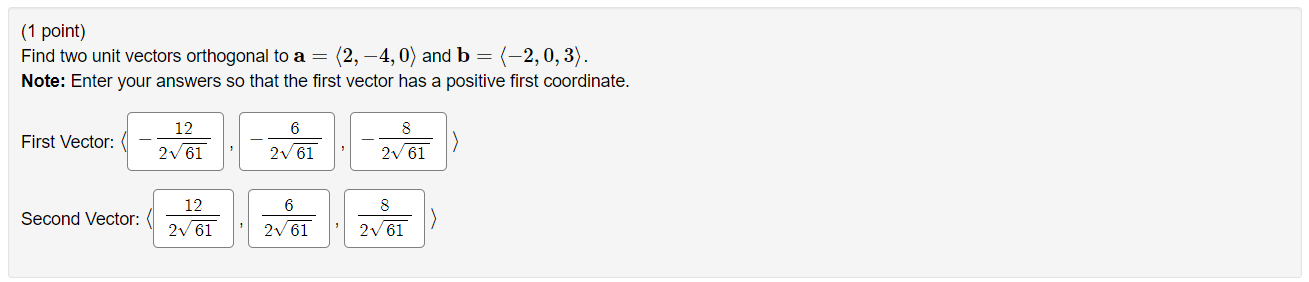### Create an Account

Already have account?

### Forgot Your Password ?

Home / Questions / (1 point) Find two unit vectors orthogonal to a = (2, -4,0) and b = (-2,0,3). Note: Enter ...

# (1 point) Find two unit vectors orthogonal to a = (2, -4,0) and b = (-2,0,3). Note: Enter your answers so that the first vector has a positive first coordinate. 6 First Vector: 12 2V 61 2005 8 261 2v

(1 point) Find two unit vectors orthogonal to a = (2, -4,0) and b = (-2,0,3). Note: Enter your answers so that the first vector has a positive first coordinate. 6 First Vector: 12 2V 61 2005 8 261 2v 61 Second Vector: 12 261 6 261 2 61Apr 15 2021 View more View Less

#### Answer (Solved)Subscribe To Get Solution